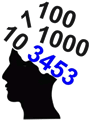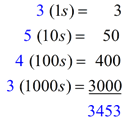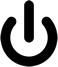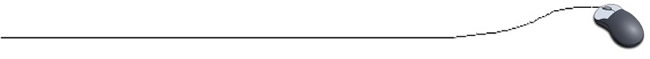Working with Number Bases

One of the skills which is useful to programmers is an understanding of the relationship between number bases.  When you understand how numbers are represented in base two (Binary), base eight (Octal), and base sixteen (Hexadecimal), you will better understand references which will be made later in this course.

The number system that we all know and love is the Decimal number system, base 10.  In our number system we utilize the digits 0, 1, 2, 3, 4, 5, 6, 7, 8, and 9.  All numbers are formed by combining these digits.

Other number bases work in the same manner only with different digits.
The Binary number system, base 2, uses only the digits 0 and 1.
The Octal number system, base 8, uses the digits 0, 1, 2, 3, 4, 5, 6, and 7.
The Hexadecimal number system, base 16, uses the
digits and letters 0, 1, 2, 3, 4, 5, 6, 7, 8, 9, A, B, C, D, E, and F.

Notice how, in each of these number systems, the number of digits contained within the system is the name of the system.  Base 2 has 2 digits, Base 10 has 10 digits, and so on.  Just be careful to remember that each system starts counting with 0.

Each system uses its digits to form all of the numbers contained within the system.  In the number system that we know and love, base 10, we are familiar with the terms ones, tens, hundreds, thousands, etc. used to describe the position a digit holds within a number.  These terms are actually words that describe powers of 10.   The positions within a number in base 10 are formed by systematically raising the number 10 to various powers.

 100 = 1 (ones)101  = 10 (tens) 102 = 100 (hundreds) 103 = 1000 (thousands)

The same process is used to create numbers in other number systems as well.  The only difference is that instead of raising 10 to a power, the new base number is used.  For example, when working in base 8, you are working with powers of 8   (80, 81, 82, 83, ...).  Base 2 works with powers of 2, base 16 works with powers of 16, and so on.

In our study of computer programming, most of our references will be made to the binary number system.  The simplicity of only dealing with two digits (0 or 1) is very appealing to a computer system.  The 0 and 1 can represent current being turned OFF or ON.  Notice how switches on computer hardware often display a 0 or a 1 to represent off or on.

 The power symbol: Toggle power switches contain a "0" and a "1" to universally indicate "Off" or "On". A single on/off button contains a combination of the "0" and the "1" as shown at the right.Now, let's go on to the next lesson page and look at
how to convert from one number base to another.Notice: These materials are free for your on-line use at this site, but are not free for the taking. Please do not copy these materials or re-post them to the Internet, as it is copyright infringement. If you wish hard-copies of these materials, refer to our subscription area, JavaMathBits.com. Help us keep these resources free. Thank you.